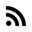# When does it worthy to use switch instead of if-else in C?

In college I asked this all the time, specially when there were a small set of choices. I read the book Computer Systems: A Programmer’s Perspective and it explains with examples what compiler does when facing either a switch or if-else statement.

I did a test compiling `gcc` with `-S` to get the assembly code:

In the red corner we have a switch statement with several entries:

``````int asm_switch(int x){
switch(x){
case 1:
x++;
break;
case 2:
x--;
break;
case 3:
x*=5;
break;
case 4:
x+=10;
break;
case 5:
x-=10;
break;
default:
x*=2;
}
return x;
}
``````

And it’s assembly code:

``````asm_switch:
.LFB3:
.cfi_startproc
pushq	%rbp
.cfi_def_cfa_offset 16
.cfi_offset 6, -16
movq	%rsp, %rbp
.cfi_def_cfa_register 6
movl	%edi, -4(%rbp)
cmpl	\$5, -4(%rbp)
ja	.L12
movl	-4(%rbp), %eax
movq	.L14(,%rax,8), %rax
jmp	*%rax
.section	.rodata
.align 8
.align 4
.L14: ;Hash Table
.text
.L13: ;x+=1
jmp	.L19
.L15: ;x-=1
subl	\$1, -4(%rbp)
jmp	.L19
.L16: ;x*=5
movl	-4(%rbp), %edx
movl	%edx, %eax
sall	\$2, %eax
movl	%eax, -4(%rbp)
jmp	.L19
.L17: ;x-=10
jmp	.L19
.L18: ;x+=10
subl	\$10, -4(%rbp)
jmp	.L19
.L12: ;x*=2
sall	-4(%rbp)
.L19:
movl	-4(%rbp), %eax
popq	%rbp
.cfi_def_cfa 7, 8
ret
.cfi_endproc
``````

As it is observed `asm_switch` has 6 cases. The compiler converts the switch statement in a hash table under the label `.L14`. As it is expected, the hash table has 6 entries, each entry correspond to each `case`. The line `jmp *%rax` uses the content of register `rax` to choose which `case` will be executed.

Now, let’s see what happens when a switch statement has few entries. In the blue corner we have a switch statement with 3 cases:

``````int short_switch( int x){
switch(x){
case 1:
x++;
break;
case 2:
x--;
break;
default:
x*=2;
}

return x;
}
``````

and it’s assembly code:

``````short_switch:
.LFB3:
.cfi_startproc
pushq	%rbp
.cfi_def_cfa_offset 16
.cfi_offset 6, -16
movq	%rsp, %rbp
.cfi_def_cfa_register 6
movl	%edi, -4(%rbp)
movl	-4(%rbp), %eax
cmpl	\$1, %eax
je	.L20
cmpl	\$2, %eax
je	.L21
jmp	.L24
.L20:
jmp	.L22
.L21:
subl	\$1, -4(%rbp)
jmp	.L22
.L24:
sall	-4(%rbp)
.L22:
movl	-4(%rbp), %eax
popq	%rbp
.cfi_def_cfa 7, 8
ret
.cfi_endproc
``````

In this case the switch statement it is compiled as a set of conditional jumps `je` (jump if it is equal to ), there is no hash table in this case. As a note, `je` checks the status of the zero flag (ZF), if it is `1` then it jumps. For example, in the line `cmpl \$1, %eax`, if the value of register `eax` is `1` then `1 -> ZF`, and the programs jumps to `.L20`.

Now let’s see the `short_switch` but implemented with if-else statements:

``````int short_ifswitch( int x){
if (x==1)
x++;
else if (x==2)
x--;
else
x*=2;

return x;
}
``````

It’s assembly code:

``````short_ifswitch:
.LFB5:
.cfi_startproc
pushq	%rbp
.cfi_def_cfa_offset 16
.cfi_offset 6, -16
movq	%rsp, %rbp
.cfi_def_cfa_register 6
movl	%edi, -4(%rbp)
cmpl	\$1, -4(%rbp)
jne	.L29
jmp	.L30
.L29:
cmpl	\$2, -4(%rbp)
jne	.L31
subl	\$1, -4(%rbp)
jmp	.L30
.L31:
sall	-4(%rbp)
.L30:
movl	-4(%rbp), %eax
popq	%rbp
.cfi_def_cfa 7, 8
ret
.cfi_endproc
``````

Of course the compiler will not generated the exact same code for `short_ifswitch`, but they are quite similar. Both of them are decoded as a combination of conditional jumps.

## Conclusion

If your have several choices `switch` statements are the way to go. But for a small set of choices it’s better, most of the time, to use `if-else` statements. In either case, for low level optimizations, always, check the assembly code by using either the `gdb` or `objdump -D`.

Get my stuff direct in your inbox